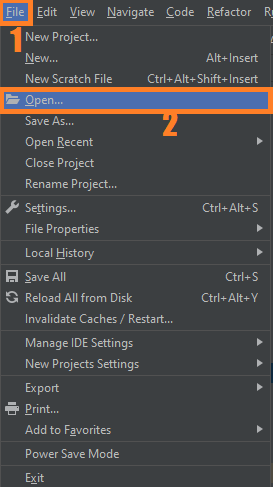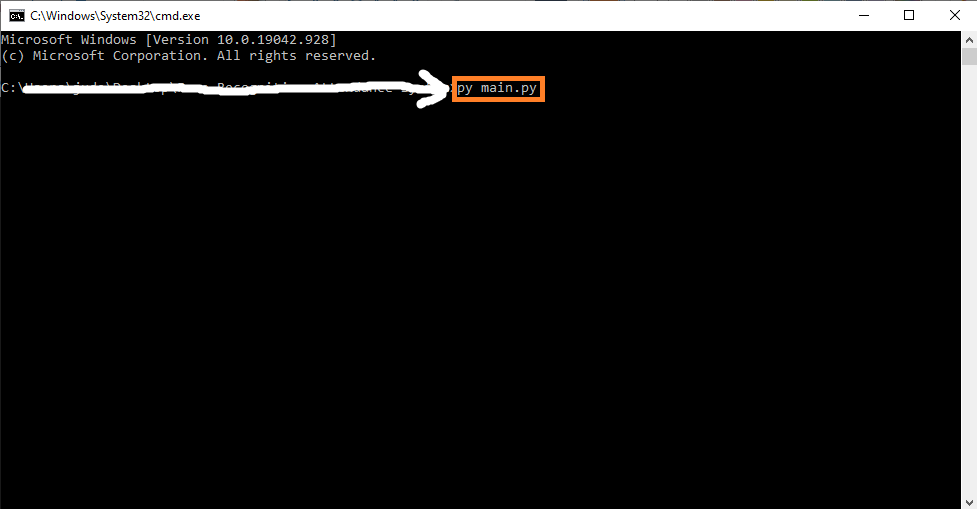# How To Make A Progress Bar in Python with Source Code

In this article you can learn the step by step process on How To Make A Progress Bar in Python, This project was created in Python Programming Language using PyCharm IDE.

The Progress Bar in Python is created using Graphical User Interface (GUI). This simple project is easy to understand and also this tutorial is good for the beginners or the students who wants to learn programming, specially Python Programming Language.

## Project Information’s

A Progress Bar tutorial teach you on How To Show Progress Bar In Python, In this code, we have declared a loop that will run 5 times. this loop will keep on updating the progress bar value with 20.

Every time loop will run, it will update the value of the Label. ws.update_idletasks() is used so that we can see the progress. Otherwise, it will quickly happen. there is a gap of 1 second every time the loop is run.

By the way if you are new to python programming and you don’t know what would be the the Python IDE to use, I have here a list of Best Python IDE for Windows, Linux, Mac OS that will suit for you. I also have here How to Download and Install Latest Version of Python on Windows.

To start on how to make a progress bar, make sure that you have installed Python 3.9 and PyCharm in your computer.

## How To Create Progress Bar in Python

Here’s the step by step process on how to create progress bar in python.

### 1. Install Libraries

First, Install all given required libraries.

``````from tkinter import *
from tkinter.ttk import Progressbar
import time``````

### 2. Use the `time.sleep()` Method

In this line of code we will use the `time.sleep()` method, to simulate a for loop that takes some time to execute.

``````def step():
for i in range(5):
pb['value'] += 20
time.sleep(1)
txt['text']=pb['value'],'%'``````

### 3. Create Progress Bar Interface

Next, let’s create an progress bar interface.

``````pb = Progressbar(
ws,
orient = HORIZONTAL,
length = 100,
mode = 'determinate'
)

pb.place(x=40, y=20)

txt = Label(
ws,
text = '0%',
bg = '#345',
fg = '#fff'

)

txt.place(x=150 ,y=20 )``````

### 4. Create a Button

Lastly, let’s create a clickable button to start functioning the progress bar.

``````Button(
ws,
text='Start',
command=step
).place(x=40, y=50)``````

### Complete Source Code

``````from tkinter import *
from tkinter.ttk import Progressbar
import time

def step():
for i in range(5):
pb['value'] += 20
time.sleep(1)
txt['text']=pb['value'],'%'

ws = Tk()
ws.title('IT SOURCECODE')
ws.geometry('200x150')
ws.config(bg='#345')

pb = Progressbar(
ws,
orient = HORIZONTAL,
length = 100,
mode = 'determinate'
)

pb.place(x=40, y=20)

txt = Label(
ws,
text = '0%',
bg = '#345',
fg = '#fff'

)

txt.place(x=150 ,y=20 )

Button(
ws,
text='Start',
command=step
).place(x=40, y=50)

ws.mainloop()``````

## Steps on how to run the project

Time needed: 5 minutes.

These are the steps on how to run Progress Bar In Python Tkinter With Source Code

First, download the given source code below and unzip the source code.• Step 2: Import the project to your PyCharm IDE.• Step 3: Run the project.

last, run the project with the command “py main.py”